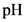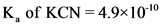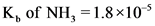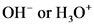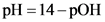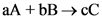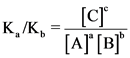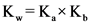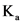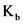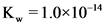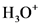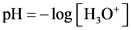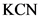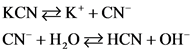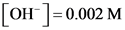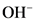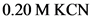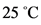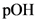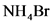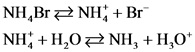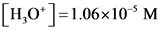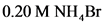# Calculate the ph of a 0.20 m solution of kcn at 25.0 ∘c

1. Calculate the pH of a 0.20 M solution of KCN at 25.0
∘C. M Express the pH numerically using two decimal places. ph= 2.Calculate the pH of a
0.20 M solution of NH4Br at 25.0 ∘C. Calculate the pH of a
0.20 M solution of NH4Br at 25.0 ∘C. Express the pH numerically using two decimal places. ph=

General guidance

Concepts and reason
of a salt is calculated by taking the help of ions produced on its dissociation in water.
According to the literature values, and.
Use the ICE table to calculate the concentration of and thereby calculating the of the solution using the formula:

Fundamentals

Acid or Base dissociation constant – It is defined as the ratio of the concentration of the products to the concentration of the reactants raised to the power of its stoichiometry in a given chemical reaction.
Consider a reaction,

Acid or Base dissociation constant for the above reaction is given as follows:

The ionization constant of water is given as follows:

Here, is acid dissociation constant and is base dissociation constant.
The value of .
of a solution is defined as the negative logarithm of the ions.
Mathematically,

Step-by-step

Step 1 of 3

Part 1

Dissociation reaction of is given as follows:

Calculate as follows:

According to the ICE table:

Apply the formula of base dissociation constant as follows:

Hence,

Dissociation reaction of in water is written. It is observed that ions are produced in the reaction. Hence, the concentration of ions are found using dissociation constant formula.
Using the formula of ionization constant of water, for the reaction is calculated thereby, calculating the concentration of ions.

Step 2 of 3

Part 1
The of solution at is 11.30.

From step 1, . Therefore, is calculated by taking its negative logarithm. Finally, of the solution is calculated by subtracting from 14.
The answer is expressed using two decimal places as asked in the question.

Step 3 of 3

Part 2

Dissociation reaction of is given as follows:

Calculate as follows:

According to the ICE table:

Apply the formula of base dissociation constant as follows:

Hence,

Part 2
The of solution at is 4.97.

Dissociation reaction of in water is written. It is observed that ions are produced in the reaction. Hence, the concentration of ions are found using dissociation constant formula.
Using the formula of ionization constant of water, for the reaction is calculated thereby, calculating the concentration of ions.
Finally, of the solution is calculated by taking the negative logarithm of ions.

Part 1
The of solution at is 11.30.

Part 2
The of solution at is 4.97.

Part 1
The of solution at is 11.30.

Part 2
The of solution at is 4.97.

pH
K of KCN 4.9×1010
K of NH 1.8x 10
ОН or H,0′ 3
pH
pH %314- рОН
аА + bВ -> сС
[ст к. К. ТАТ [В [АT[В]
a
K.
К,
K 1.0×10
pH
Н,О
pH-log H,0
KCN
K of KCN 4.9×1010
KCN
KCN KCN CN H0 HCN +OH’
К,
К, К. К, 1.0×101 4.9×10 10 2×10
CN
HCN
ОН
0.2 M
(0.2- х)М
[HCNOH [CN 2×10=T02-x)M xxx x+2x10x M-4×10 M 0 = 0.002 M
[OH0.002 M
KCN
ОН
ОН
К,
ОН
pOH-log [OH] –log 0.002 =2.70 M
PH 3D14- рОН =14-2.7 =11.30
[OH0.002 M
РОН
pH
РОН
K of NH 1.8x 10
NH,Br
NH4BrN Br NHH NH, H,0*
K.
K KxK К. к, К» 1.0×1014 1.8×10 =5.6×1010
NH 4
NH 3
Н,О
0.2 M
(0.2- х)М
[NH,H,O к, NH; хXX 5.6×10-10 (0.2-к)м * +(56х10°)х-(1.12×10″)-0 x= 1.06x 10 M
Но]-1.06х10* м
pH 3D- log| H,О — log (1.06×103) =4.97 M
NH,Br
Н,О
Н,О
K.
Н,О
pH
Н,О
pH
0.20 М КCN
25 “C
pH
0.20 М КCN
25 “C
pH
0.20 М КCN
25 “C
pH
0.20 М КCN

 I 0 0 C –x +x +x E x x
 I 0 0 C –x +x +x E x x